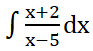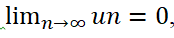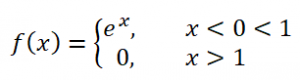# Pokhara University|| Fall 2016|| Mathematics||

Fall 2016  Business Mathematics

This is the question set along with answers of Fall 2016  Business Mathematics, which was taken by Pokhara University.

POKHARA UNIVERSITY

Fall 2016  Business Mathematics

 Level:  Bachelor Semester – Fall Year: 2016 Program: BCIS Full Marks: 100 Course: Mathematics II Pass Marks: 45 Time:3hrs Candidates are required to give their answers in their own words as far as practicable. The figures in the margin indicate full marks.

Section “A”

Very Short Answer Questions

 Attempt all the questions. 10×2 1 Evaluate2 2 Show that2 3 Test whether the seriesis convergent. 2 4 Prove that u = x2 – y2 is harmonic 2 5 Solve the differential equation: y dx – x dy =  x2y dx 2 6 Express in the polar form √ 3 + i 2 7 Integrate2 8 Define a periodic function with an example. 2 9 Find the period of the function  f(x)=cosnx 2 10 Solve2

Section “B”

 Attempt any six questions 6×10 11 Integrate any two of the following.12 a)  Find the volume of solid generated by revolving about the x – axis, the areas bounded by the curve y=5x – x2 and the lines; x = 0, x = 5. Find the arc length of the curve,  y=2/3 (x+1)2/3,0≤x  ≤2 13 Solve the following differential equations: a)b)14 a)    Solve:Prove that the necessary condition for the convergence of an infinite series ∑un isbut it is not sufficient. 15 a)    Test for the convergence series of theb)    Find  Fourier sine and cosine integral of the function16 a)    Find the Fourier series of the functionFind the Fourier series of the function. 17 Find the harmonic conjugate and corresponding analytic function ofWhat are the necessary conditions for a function of complex variables to be analytic? Show that the function f(z)=is analytic.

Section “C”

 Case Analysis 18 a)    Letbe defined and continuous in some neighborhoods of a point  Z=x+iy and differentiable at Z itself. Then at that point , the first order Partial derivatives of u and v exist and satisfy the Cauchy Riemann equationprove. State and prove P-series test.

You may also like Pokhara University||2015 Fall Business Mathematics ||

Do follow us on Online Notes Nepal